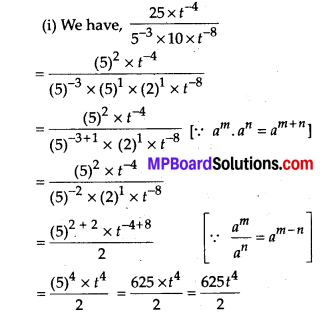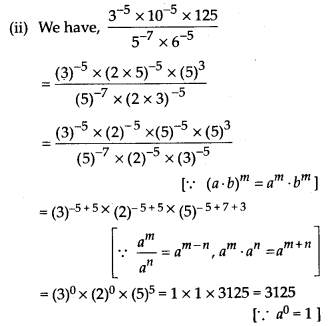# MP Board Class 8th Maths Solutions Chapter 12 Exponents and Powers Ex 12.1

## MP Board Class 8th Maths Solutions Chapter 12 Exponents and Powers Ex 12.1

Question 1.
Evaluate
(i) 3-2
(ii) (-4)-2
(iii) 
Solution: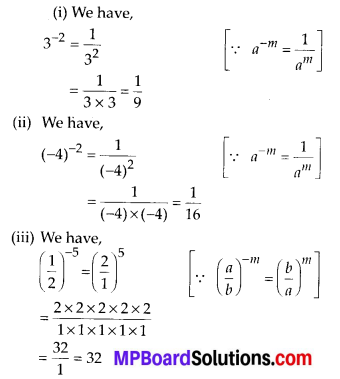Question 2.
Simplify and express the result in power notation with positive exponent.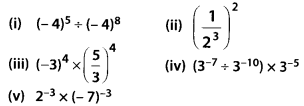Solution: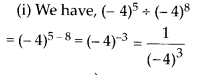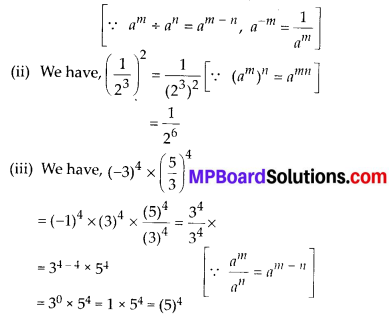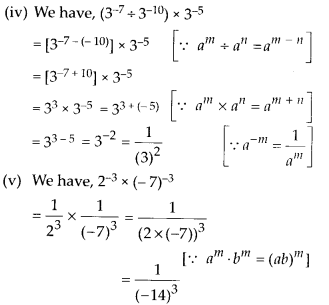Question 3.
Find the value of
(i) (30 + 4-1) × 22
(ii) (2-1 × 4-1) ÷ 2-2
(iii) $$\left(\frac{1}{2}\right)^{-2}+\left(\frac{1}{3}\right)^{-2}+\left(\frac{1}{4}\right)^{-2}$$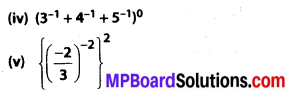Solution: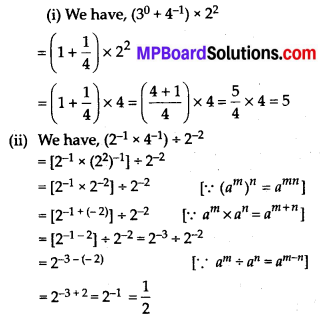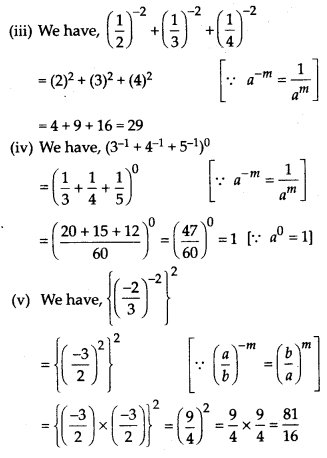Question 4.
Evaluate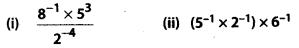Solution: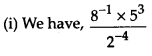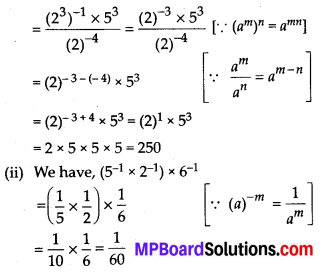Question 5.
Find the value of m for which 5m ÷ 5-3 = 55.
Solution:
We have, 5m ÷ 5-3 = 55
⇒ 5m-(-3) = 55 [∵ am + an =am + n]
⇒ 5m + 3 = 55
⇒ m + 3 = 5 [∵ am = an ⇒ m = n]
⇒ m = 5 – 3 = 2Question 6.
Evaluate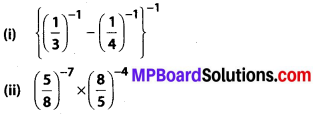Solution: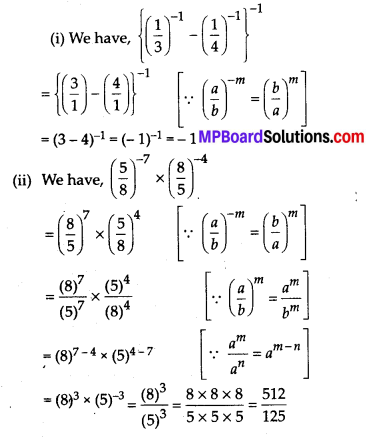Question 7.
Simplify.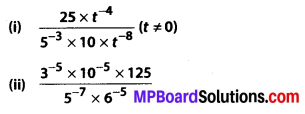Solution: